# Texas Go Math Grade 4 Lesson 10.4 Answer Key Place the First Digit

Refer to our Texas Go Math Grade 4 Answer Key Pdf to score good marks in the exams. Test yourself by practicing the problems from Texas Go Math Grade 4 Lesson 10.4 Answer Key Place the First Digit.

## Texas Go Math Grade 4 Lesson 10.4 Answer Key Place the First Digit

Essential Question

How can you use place value to know where to place the first digit in the quotient?
Answer: place value is the value of the digit in its place.

Unlock the Problem

Jaime took 144 photos on a digital camera. The photos are to be placed equally in 6 photo albums. asked to find. How many photos will be in each album?

• Underline what you are
• Circle what you need to use.

We need to find the number of photos will be there in each album.

Example Divide. 144 ÷ 6

STEP 1 Use place value to place the first digit.
Look at the hundreds in 144.
1 hundred cannot be shared among 6 groups without regrouping.
Regroup 1 hundred as 10 tens.
Now there are _________ tens to share among 6 groups.
The first digit of the quotient will be in the ___________ place.The first digit of the quotient is in tens place.

STEP 2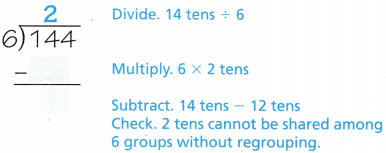6 x 2 = 12

So, 14 – 12 = 2

Math Idea: After you divide each place, the remainder should be less than the divisor.

STEP 3 Divide the ones.
Regroup 2 tens as 20 ones.
Now there are _________ ones to share among 6 groups.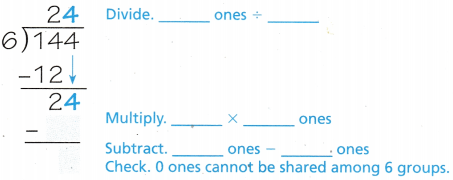So, there will be _________ photos in each album.So, there are 24 photos in each album.

Math Talk

Mathematical Processes

If Jamie had 146 photos, then

146/6Quotient : 24

Remainder : 2

So, The answer in remainder changes if Jacob have 146 photos.

Share and Show

There are 452 pictures of dogs in 4 equal groups. How many pictures are in each group? Explain how you can use place value to place the first digit in the quotient.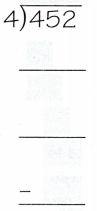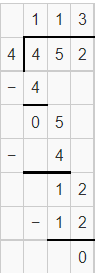Quotient = 113

Remainder = 0

Divide.

Question 2.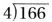Quotient = 041

Remainder= 2

Question 3.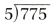Quotient = 155

Remainder= 0

Math Talk

Mathematical Processes
Explain how you placed the first digit of the quotient in Exercise 2.

We can place the first digit in the first place.

Problem Solving

Practice: Copy and Solve Divide.

Question 4.
516 ÷ 2Quotient = 258

Remainder= 0

Go Math Lesson 10.4 4th Grade Place The First Digit Question 5.
516 ÷ 3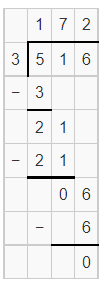Quotient = 172

Remainder= 0

Question 6.
516 ÷ 4Quotient = 129

Remainder= 0

Question 7.
516 ÷ 5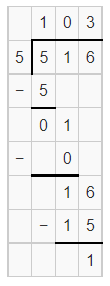Quotient = 103

Remainder= 1

Question 8.
H.O.T. Look hack at your answers to Exercises 4-7. What happens to the quotient when the divisor increases? Explain.

The value of remainder increases when the value of divisor increases.

Question 9.
Multi-Step Reggie has 192 pictures of animals. He wants to keep half and then divide the rest equally among three friends. How many pictures will each friend get?
(A) 96
(B) 32
(C) 48
(D) 14

Total number of animal pictures Reggie have = 192

Given, he kept half of them

Which means,

192/2

= 96

Now, 96 animal pictures are shared equally among 3 friends.

So, 96/3 =

32

Therefore, each friend gets 32 pictures.

H.O.T. Multi-Step There are 146 students, 5 teachers, and 8 chaperones going to the theater. To reserve their seats, they need to reserve entire rows. Each row has 8 seats. How many rows must they reserve?
(A) 20
(B) 18
(C) 19
(D) 58

Given,

Number of students = 146

Number of teachers = 5

Number of chaperones = 8

Total number of people = 146+5+8

= 159

Also give, the number of people for each row = 8

So, 159/8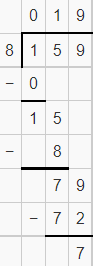Therefore, They must reserve 19 rows.

Unlock the Problem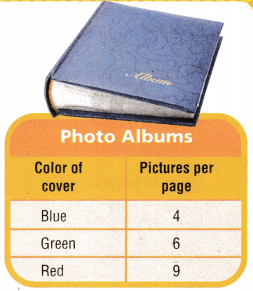Question 11.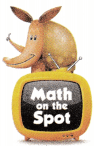H.O.T. Multi-Step Nan wants to put 234 pictures in an album with a blue cover. How many full pages will she have in her album?

Number of pictures Nan have = 234

Pictures per blue page = 4

So, 234/4Therefore, 58 full pages are there in her album.

a. What do you need to find?

We need to find the number of full pages in Nan album

b. Communicate How will you use division to find the number of full pages?

We can find the number of full pages by dividing number of pictures Nan have by the number of pictures for blue page.

c. Show the steps you will use to solve the problem.d. Complete the following sentences.
Nan has ________ pictures.
She wants to put the pictures in an album with pages that each hold ________ pictures.
She will have an album with ________ full pages and ________ pictures on another page.

Nan has 234 pictures.
She wants to put the pictures in an album with pages that each hold 4 pictures.
She will have an album with 58 full pages and 4 pictures on another page.

Question 12.
Apply Juan wants to put his 672 pictures in an album with a green cover. how many full pages will he have in his album?

For green color, pictures per page = 6

Number of pictures Juan in an album = 672Therefore, he have 112 full pages in his album.

Go Math Answer Key Grade 4 Lesson 10.4 Place The First Digit Question 13.
H.O.T. Multi-Step Talla has 162 pictures to put in a photo album. If she wants only full pages, which color albums could she use?

Number of pictures Talla have = 162

For blue color, number of pictures per page = 4

So,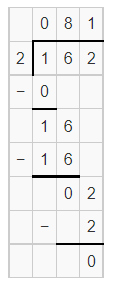Remainder = 0 Which means there are only full pages.

Therefore, She should use blue color.

Question 14.
Danny makes a website to showcase images of unusual manhole covers. He takes 264 pictures for the site. Danny places 8 pictures on each page of the site. How many pages of pictures does Danny’s site have?
(A) 33 pages
(B) 330 pages
(C) 3 pages
(D) 30 pages

Number of pictures Danny takes = 264

Number of pictures he kept on each page of the site = 8

Now,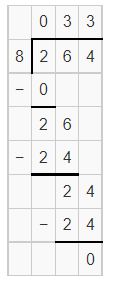Therefore, 33 pages of pictures does Danny’s site have

Question 15.
Debi celebrates her birthday with friends at an arcade. Debi’s parents want to share 300 tokens equally among the 9 friends. Debi uses division to find how many tokens each person gets. In which place is the first digit of the quotient?
(A) thousands
(B) hundreds
(C) tens
(D) ones

Total number of token = 300

Number of friends = 9

Also given, Debi’s parents give each person the same number of tokens.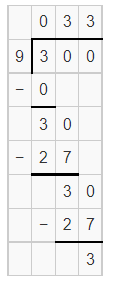Quotient = 033

Remainder = 3

The 0 is in hundreds place, 3 is in tens place.

Question 16.
Multi-Step Debi’s parents want to share 300 tokens equally among the 9 friends. After Debi’s parents give each person the same number of tokens, how many tokens are left over?
(A) 0 tokens
(B) 2 tokens
(C) 1 token
(D) 3 tokens

Total number of token = 300

Number of friends = 9

Also given, Debi’s parents give each person the same number of tokens.Remainder = 3

Therefore, the number of tokens left = 3

TEXAS Test Prep

Question 17.
Kat wants to put 485 pictures in an album with a red cover. There are 9 pictures per page. She uses division to find out how many full pages she will have. In which place is the first digit of the quotient?
(A) thousands
(B) hundreds
(C) tens
(D) ones

The number of pictures Kat wants to keep in album = 485

Number of pictures per page = 9

So,The first digit in quotient is in tens place.

### Texas Go Math Grade 4 Lesson 10.4 Homework and Practice Answer Key

Question 1.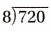Quotient = 090

Remainder = 0

Question 2.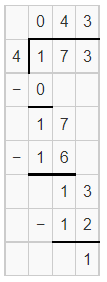Quotient = 043

Remainder = 1

Question 3.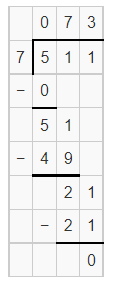Quotient = 073

Remainder = 0

Question 4.Quotient = 046

Remainder = 1

Question 5.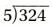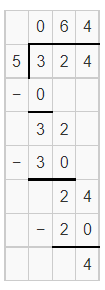Quotient = 064

Remainder = 4

Question 6.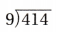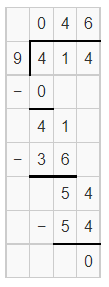Quotient = 046

Remainder = 0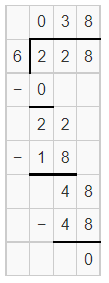Quotient = 038

Remainder = 0

Question 8.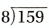Quotient = 019

Remainder = 7

Question 9.
428 ÷ 2 ____________Quotient = 214

Remainder = 0

Question 10.
428 ÷ 3 ____________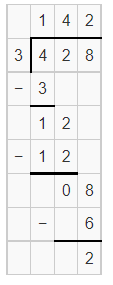Quotient = 142

Remainder = 2

Question 11.
428 ÷ 4 ____________Quotient = 107

Remainder = 0

Question 12.
428 ÷ 5 ____________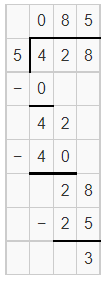Quotient = 107

Remainder = 0

Problem Solving

Question 13.
Camp Mesquite will provide 4 buses for 212 campers. If each bus carries the same number of campers, how many campers will ride in each bus?

Total number of campers = 212

Number of buses = 4

If each bus carries the same number of campers,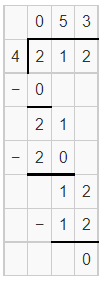Therefore, 53 campers will ride in each bus.

Question 14.
The garden center received a shipment of 132 daisies. If each table displays the same number of daisies, how many daisies will be placed on each of 3 tables?

Number of daisies shipped = 132

Number of tables = 3

If each table displays the same number of daisies,

Now,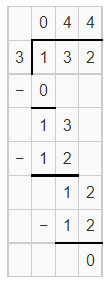Therefore, 44 daisies will be placed on each of 3 tables.

Lesson Check

Question 15.
Lauren collected 532 pennies in a donation box. She wants to divide the pennies equally into 4 containers. Lauren uses division to find how many pennies to put into each container. In which place is the first digit of the quotient?
(A) thousands
(B) hundreds
(C) tens
(D) ones

The number of pennies Lauren collected = 532

Number of boxes = 5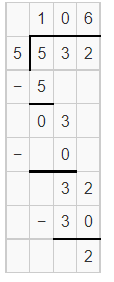The digit of the quotient is in Hundreds place.(1)

Question 16.
Mark opens a box of 500 cups for the football concession stand. Mark uses division to divide the cups equally among windows. In which place is the first digit of the quotient?
(A) ones
(B) tens
(C) hundreds
(D) thousands

Question 17.
Mrs. Samson bought 294 craft sticks for a class project. She divides her class into 7 groups and gives each group an equal number of sticks. If she uses all of the sticks, how many will each group receive?
(A) 40
(B) 43
(C) 41
(D) 42

Number of sticks Samson bought to the class = 294

Number of groups she divides her class = 7

Also given, She uses all the sticks in equal number.

So,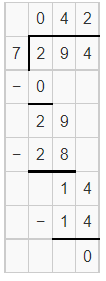Therefore, Each group receive 42 sticks.

Question 18.
Cassie read 483 pages of her hook in one week. if she read the same number of pages each day, how many pages did she read in 1 day?
(A) 78
(B) 79
(C) 69
(D) 68

Total number of pages Cassie read  = 483

Number of days in a week = 7

If she read the same number of pages each day,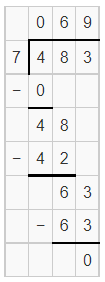Therefore, Cassie read 69 pages in 1 day.

Question 19.
Multi-Step Mr. Parsons bought 293 apples to make pies for his shop. Six apples are needed for each pie. If Mr. Parsons makes the most apple pies possible, how many apples will be left over?
(A) 5
(B) 1
(C) 3
(D) 6

Number of apples Parsons bought = 293

Number of apples required to make 1 apple pie = 6

So, 293/6 = 48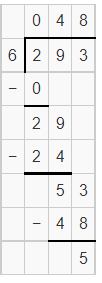Now, 6 x 48 = 288

So, Total – Number of apples required = leftover

293-288 = 5

Therefore, the number of apples left = 5.

Question 20.
Multi-Step At an art school, 112 students are in 4 equal-sized drawing classes. There are 120 students in 5 equal-sized painting classes. I low many more students are in one drawing class than are in one painting class?
(A) 28
(B) 5
(C) 25
(D) 4

Total number of students in 4 equal sized drawing class = 112

Now, 112/4

= 28

Total number of students in 5 equal sized drawing class = 120

Now, 120/5

= 24

Now, The number of more students = 28 – 24

= 4

Therefore,4 students are in one drawing class than are in one painting class.

Scroll to Top